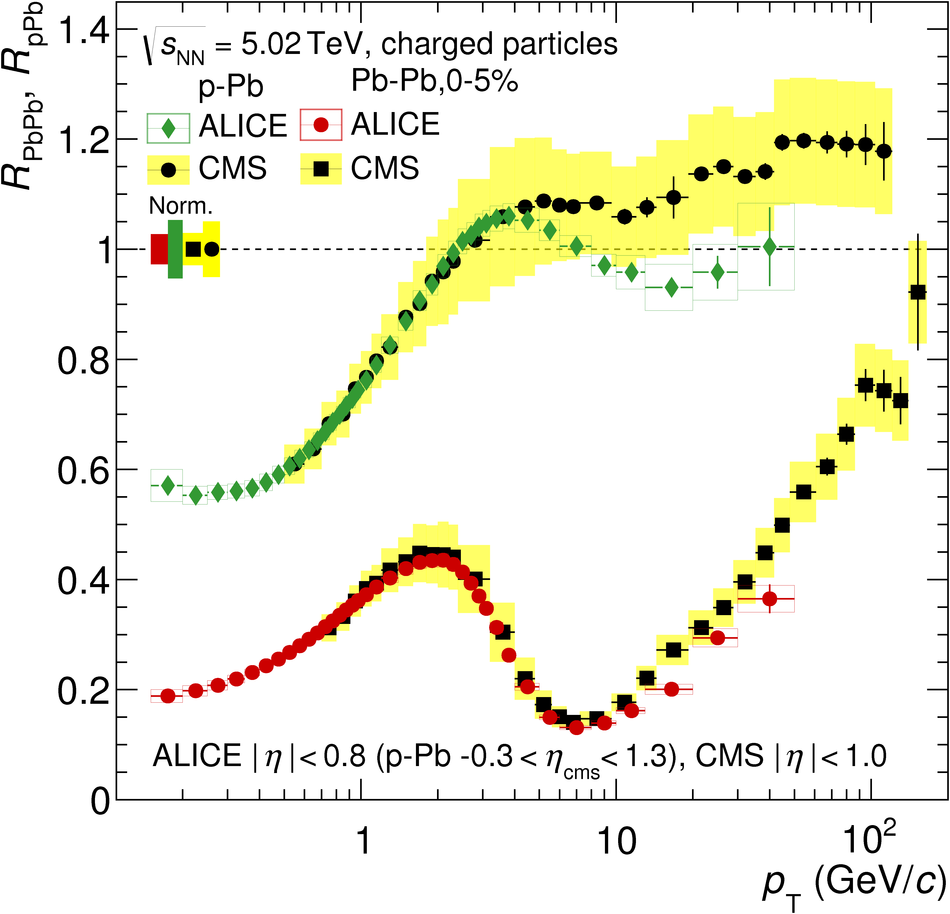Transverse momentum spectra and nuclear modification factors of charged particles in pp, p-Pb and Pb-Pb collisions at the LHC

We report the measured transverse momentum ($p_{\rm T}$) spectra of primary charged particles from pp, p-Pb and Pb-Pb collisions at a center-of-mass energy $\sqrt{s_{\rm NN}} = 5.02$ TeV in the kinematic range of $0.15<~p_{\rm T}<~50$ GeV/$c$ and $|\eta|<~ 0.8$. A significant improvement of systematic uncertainties motivated the reanalysis of data in pp and Pb-Pb collisions at $\sqrt{s_{\rm NN}} = 2.76$ TeV, as well as in p-Pb collisions at $\sqrt{s_{\rm NN}} = 5.02$ TeV, which is also presented. Spectra from Pb-Pb collisions are presented in nine centrality intervals and are compared to a reference spectrum from pp collisions scaled by the number of binary nucleon-nucleon collisions. For central collisions, the $p_{\rm T}$ spectra are suppressed by more than a factor of 7 around 6-7 GeV/$c$ with a significant reduction in suppression towards higher momenta up to 30 GeV/$c$. The nuclear modification factor $R_{\rm pPb}$, constructed from the pp and p-Pb spectra measured at the same collision energy, is consistent with unity above 8 GeV/$c$. While the spectra in both pp and Pb-Pb collisions are substantially harder at $\sqrt{s_{\rm NN}} = 5.02$ TeV compared to 2.76 TeV, the nuclear modification factors show no significant collision energy dependence. The obtained results should provide further constraints on the parton energy loss calculations to determine the transport properties of the hot and dense QCD matter.

Figures

Figure 1

 Left: Combined tracking efficiency and acceptance as a function of \pT for different particle species and the sum of all, obtained in Monte Carlo simulations of pp collisions at $\s = 5.02$~TeV with PYTHIA 8 (Monash 2013 tune). For $\pt > 1$~GeV/$c$ parameterizations are shown. The relative systematic uncertainties on parameterizations are small ($< 0.2\%$) and are not shown. The statistical uncertainties for $\pt < 1$~GeV/$c$ are smaller than the symbol size ($< 0.5\%$). Right: The relative particle abundances as a function of \pt in Monte Carlo (open symbols, for $\s = 5.02$~TeV) and in data (full symbols, for $\s = 7$~TeV). The data are extrapolated beyond the range of the measurements (see description in the text). The statistical and systematic uncertainties (combined $< 1.6\%$) are not shown.Figure 2

 Left: Combined tracking efficiency and acceptance as a function of \pT for pp, p--Pb, central (0-5\%) and peripheral (70--80\%) Pb--Pb collisions determined using Monte Carlo simulations and a reweighting method (see text for details). For better visibility, the curves for p--Pb and Pb--Pb are offset by the indicated values. The effect of the reweighting on the efficiency corrections is shown in the bottom panel. The systematic uncertainties of the reweighting ($< 2.4\%$) are not shown Right: Contamination from secondary particles estimated from Monte Carlo simulations and from the impact parameter fits in data (see text for details). The effect of the reweighting of secondary particles is shown in the bottom panel. The systematic uncertainties on the scaling factors ($< 20\%$) are not shown.Figure 3

 Transverse momentum distributions of primary charged particles in $|\eta|< 0.8$ in nine centrality intervals in Pb--Pb collisions at \snn = 2.76 (left) and 5.02 TeV (right) (scale factors as indicated are used for better visibility). The data for pp collisions, obtained scaling the cross section by $\sigma^{\rm NN}_{\rm inel}$, and NSD p--Pb at $\snn~=~5.02$~TeV are also shown. The relative systematic uncertainties are shown in the lower panels for various datasets; these do not contain the normalization uncertainty.Figure 4

 Comparison of the charged-particle transverse momentum spectra measured in pp collisions to PYTHIA 8 (Monash-2013 tune) and EPOS model calculations at \s = 2.76 (top) and 5.02 TeV (bottom). The statistical uncertainties of the data and model calculations are added in quadrature. The boxes represent systematic uncertainties of the data.Figure 5

 Ratio of transverse momentum spectra at \snn = 5.02 and \snn = 2.76 TeV for Pb--Pb collisions, for nine centrality classes, and in pp collisions (repeated in each panel). The relative normalization uncertainties due to the centrality determination are indicated for each centrality class. For the pp spectrum, the relative normalization uncertainty is $\pm 3\%$.Figure 6

 The transverse momentum dependence of the nuclear modification factor measured in Pb--Pb collisions, for nine centrality classes. The new data at \snn = 5.02 (full symbols) are compared to the reanalyzed data at \snn = 2.76 TeV (open symbols). The normalization uncertainties are shown as boxes around unity.Figure 7

 Left: Nuclear modification factors measured by ALICE in central (0--5\%) and peripheral (70--80\%) Pb--Pb collisions and in p--Pb collisions at $\snn = 5.02$ TeV. Right: A comparison of the nuclear modification factors for central (0-5\%) Pb--Pb and p--Pb collisions measured by ALICE and CMS . In both figures, the \pt-dependent systematic uncertainties are shown as boxes around data points. The normalization uncertainties are shown as boxes around unity.Figure 8

 The charged-particle nuclear modification factor measured in the 0--5\% most central Pb--Pb collisions at $\snn = 5.02$~TeV in comparison to model predictions (lower panel) and (upper panel). The red boxes around data points represent \pt dependent systematic uncertainties. The normalization uncertainty of the data ($\pm 2.7\%$) is not part of the uncertainties of the plotted data points